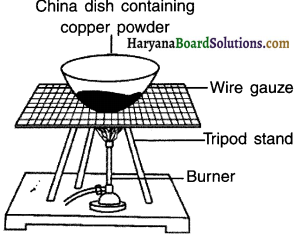# HBSE 10th Class Science Solutions Chapter 1 Chemical Reactions and Equations

Haryana State Board HBSE 10th Class Science Solutions Chapter 1 Chemical Reactions and Equations Textbook Exercise Questions and Answers.

## Haryana Board 10th Class Science Solutions Chapter 1 Chemical Reactions and Equations

HBSE 10th Class Science Chemical Reactions and Equations Textbook Questions and Answers

Question 1.
Which of the statements about the reaction below are incorrect?
2 PbO(s) +C(s) →2Pb (s)+CO2(g)
(b) Carbon dioxide is getting oxidized.
(c) Carbon is getting oxidized.
(d) Lead oxide is getting reduced.
(i) (a) and (b)
(ii) (a) and (c)
(iii) (a), (b) and (c)
(iv) all
(i) (a) and (b)Question 2.
Fe2O3+2Al → Al2O3, +2Fe
The above reaction is an example of a ………..
(a) combination reaction.
(b) double displacement reaction.
(c) decomposition reaction.
(d) displacement reaction.
(d) displacement reaction.
Reason: Aluminium (Al) displaces iron (Fe).

Question 3.
What happens when dilute hydrochloric acid is added to iron fillings? Tick the correct answer.
(a) Hydrogen gas and iron chloride are produced
(b) Chlorine gas and iron hydroxide are produced
(c) No reaction takes place
(d) Iron salt and water are produced
(a) Hydrogen gas and iron chloride are produced.

Question 4.
What is a balanced chemical equation? Why should chemical equations be balanced?
The process of adding atoms to the elements on one or both sides i.e. reactant and product side so that the numbers of atoms of elements on each side becomes equal is known as balancing a chemical equation. Such an equation is called a balanced equation.

Need of balancing:
As per the universal law of conservation of mass, mass can neither be created nor destroyed in a chemical reaction. In other words, the total mass of the elements present in the products of chemical reaction should be equal to the total mass of the elements present in the reactants.Now atoms have mass. Hence, in order to fulfill the above condition we need to make sure that the number of atoms in each element remains same on both the sides.
(a) Mg + O2 → MgO Unbalanced. Hence, wrong chemical equation
(b) 2Mg + O2 → 2MgO Balanced. Hence, correct chemical equation

Question 5.
Translate the following statements into chemical equations and then balance them.
(a) Hydrogen gas combines with nitrogen to form ammonia.
(b) Hydrogen sulphide gas burns in air to give water and sulphur dioxide.
(c) Barium chloride reacts with aluminium sulphate to give aluminium chloride and a precipitate of barium sulphate.
(d) Potassium metal reacts with water to give potassium hydroxide and hydrogen gas.
(a) 3H2(g) + N2(g) → 2NH3(g)
(b) 2H2S(g) + 3O2(g) → 2SO2(g) + 2H2O(l)
(c) 3BaCl2(aq) + Al2(SO4)3(aq) → 2AlCl3(aq) + 3BaSO4(s)
(d) 2K(s) + 2H2O(l) → 2KOH(aq) + H2(g)

Question 6.
Balance the following chemical equations.
(a) HNO3 + Ca(OH)2 → Ca(NO3)2 + H2O
(b) NaOH + H2SO4 → Na2SO4 + H2O
(c) NaCl + AgNO3 → AgCl + NaNO3
(d) BaCl2 + H2SO4 → BaSO4 + HCl
(a) 2HNO3 + Ca(OH)2→ Ca(NO3)2 + 2H2O
(b) 2NaOH + H2SO4 → Na2SO4 + 2H2O
(c) NaCl + AgNO3 → AgCl + NaNO3
(d) BaCl2 + H2SO4 → BaSCU + 2HCl

Question 7.
Write the balanced chemical equations for the following reactions.
(a) Calcium hydroxide + Carbon dioxide → Calcium carbonate + Water
(b) Zinc + Silver nitrate → Zinc nitrate + Silver
(c) Aluminium + Copper chloride → Aluminium chloride + Copper
(d) Barium chloride + Potassium sulphate → Barium sulphate + Potassium chloride
(a) Ca(OH)2 + CO2 → CaCO3 + H2O
(b) Zn + 2AgNO3 → Zn(NO3)2 + 2Ag
(c) 2Al + 3CuCl3 → 2AlCl3 + 3Cu
(d) BaCl2 + K2SO4 → BaSO4 + 2KClQuestion 8.
Write the balanced chemical equation for the following and identify the type of reaction In each case.
(a) Potassium bromide(aq) + Barium iodide(aq) → Potassium iodide(aq) + Barium bromide(s)
(b) Zinc carbonate(s) → Zinc oxide(s) + Carbon dioxide(g)
(c) Hydrogen(g) + Chlorine(g) → Hydrogen chloride(g)
(d) Magnesium(s) + Hydrochloric acid(aq) → Magnesium chlorlde(aq) + Hydrogen(g)
(a) 2KBr(aq) + Bal(aq) → 2Kl(aq) + BaBr2(s) — Type: Double displacement reaction
(b) ZnCO3(s) → ZnO(s) + CO2(g) — Type: Decomposition reaction
(c) H(g) + Cl(g) → 2HCl(g) — Type: Combination reaction
(d) Mg(s) + 2HCl(aq) → MgCl2(aq) + H2(g) — Type: Displacement reaction

Question 9.
What does one mean by exothermic and endothermic reactions? Give examples.
A chemical reaction in which heat is released (evolved) along with the formation of products is called an exothermic reaction. (Note: Exothermic is not a major type of reaction unlike combination reaction, displacement reaction, etc.)

Example:
(i) Burning of natural gas –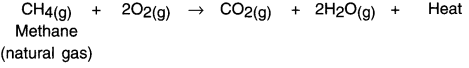(ii) Respiration
(iii) Decomposition of vegetable matter into compost.

Endothermic reaction:
A reaction in which heat is absorbed or say required is called endothermic reaction.
Example: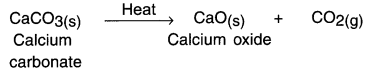When calcium carbonate is supplied heat, calcium oxide and carbon dioxide are formed.Question 10.
Why is respiration considered an exothermic reaction? Explain.

• Food gives us energy which helps us to survive.
• When we eat food, our body starts the digestion process. During digestion, the food gets broken into simple substances. For example, carbohydrate present in rice, potato, etc. breaks down to form glucose.
•  The glucose then combines with oxygen present in the body cells and provides energy. This reaction is known as respiration.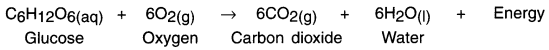• Since heat energy is released during respiration, it is known as exothermic reaction.

Question 11.
Why are decomposition reactions called the opposite of combination reactions? Write equations for these reactions.
In a decomposition reaction, a single compound breaks down to produce two or more simpler substances.
For example, mercuric oxide on heating decomposes to mercury and oxygen.
2HgO → 2Hg + O2

In a combination reaction, two or more substances simply combine to form a new substance. For example, magnesium combines with oxygen to give magnesium oxide. This process is opposite of decomposition reaction.
2Mg + O2 → 2MgO

Hence, decomposition reactions are called opposite of combination reaction.Question 12.
Write one equation each for decomposition reactions where energy is supplied in the form of heat, light or electricity.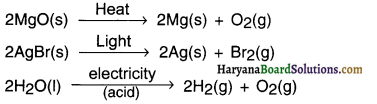Question 13.
What is the difference between displacement and double displacement reactions? Write equations for these reactions.
In displacement reactions, a more reactive metal displaces a less reactive metal from its solution
while in double displacement reactions, two reactants in solution exchange their ions. For example,
1. Fe(s) + CuSO4(aq) → Cu(s) + FeSO4(aq)
This is a displacement reaction because iron displaces copper from its solution.

2. AgNO3(aq) + NaCl(aq) → AgCl(s) + NaNO3
This is a double displacement reaction. In this reaction silver nitrate and sodium chloride exchange CF and NO ions.

Question 14.
In the refining of silver, the recovery of silver from silver nitrate solution involved displacement by copper metal. Write down the reaction involved.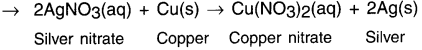Question 15.
What do you mean by a precipitation reaction? Explain by giving examples.
When two reactants in a solution react and one or more of the products formed is insoluble or forms a precipitate, the reaction is called a precipitation reaction.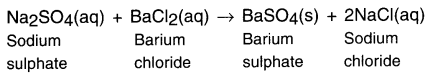When the aqueous solution of sodium sulphate reacts with aqueous solution of barium chloride a white precipitate of BaSO4 is formed.Question 16.
Explain the following in terms of gain or loss of oxygen with two examples each.
(a) Oxidation, (b) Reduction
(a) Oxidation: A chemical reaction in which a substance gains oxygen (or loses hydrogen) is called oxidation.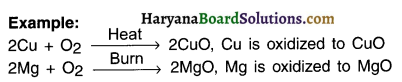(b) Reduction: A chemical reaction in which a substance loses oxygen (or gains hydrogen) is called reduction.
Example:
ZnO + O2 → Zn + CO (Here ZnO is reduced to Zn)
CuO + H2 → Cu+ H2O (Here CuO is reduced to Cu)

Question 17.
A shiny brown coloured element ‘X’ on heating in air becomes black in colour. Name the element ‘X’ and the black coloured compound formed.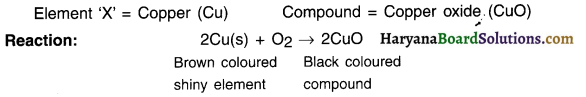Question 18.
When iron objects come in contact of moist air they get corroded.
By applying paint the iron surface gets a protective layer. This layer of paint works as a barrier and so the surface does not comes in direct contact of moist air. This prevents the corrosion of iron.

Question 19.
Oil and fat containing food items are flushed with nitrogen. Why?
1. Food items such as snacks like pun, chakri, etc. containing oil react with oxygen and become rancid. They then develop bad taste and smell.

2. To prevent them from getting spoiled they are flushed with unreactive gases such as nitrogen.Question 20.
Explain the following terms with one example each (a) Corrosion, (b) Rancidity.
(a) Corrosion

• When a metal comes in contact with humid air, moisture or a chemical such as acid, the surface of metal starts getting eaten up. This is called corrosion.
• Corrosion is mainly caused by the oxidation of metals in the humid air. Rusting of iron is the most common form of corrosion.
• Due to corrosion, silver ornaments become black and a green coating gets deposited on copper vessels.
• Corrosion damages vehicles, bridges, iron rails, ships and other metal structures.
• Corrosion of iron is a serious problem. It causes huge sum to be spent on maintaining iron structures and replacing corroded parts.

(b) Rancidity.

• Oxidation affects food that contains fats and oils.
• When food items (such as snacks like pun, chakni, chavana, etc) prepared using fat and oils are kept for longer period, they develop an unpleasant smell and taste. We then say that the food item has become rancid.
• One can reduce the rate of rancidity by keeping the food items in air-tight containers. This, slows down oxidation of food.
• Packets of chips are flushed with nitrogen to prevent chips from becoming rancid.

HBSE 10th Class Science Chemical Reactions and Equations InText Activity Questions and Answers

Textbook Page no – 6

Question 1.
Why should a magnesium ribbon be cleaned before burning in air?
Magnesium ribbon reacts with oxygen present in air to form a protective and inert layer of magnesium oxide on its surface. This layer is unreactive and prevents the ribbon from burning. Hence, it needs to be cleaned with sandpaper before burning in air.Question 2.
Write the balanced equation for the following chemical reactions:
(i) Hydrogen + Chlorine → Hydrogen chloride
(ii) Barium chloride + Aluminium sulphate → Barium sulphate + Aluminium chloride
(iii) Sodium + Water → Sodium hydroxide + Hydrogen
(i) H2 + Cl2 → HCl
H2 + Cl2 → 2HCl

(ii) BaCl2 + Al2(SO4)3 → BaSO4 + AlCl3
∴ 3BaCl2 + Al2(SO4)3 -4 3BaSO4 + 2AlCl3

(iii) Na + H2O → NaOH + H2
2Na + 2H2O → 2NaOH + H2

Question 3.
Write a balanced chemical equation along with symbols for the following reactions:
1. Solutions of barium chloride and sodium sulphate in water react to give insoluble barium sulphate and the solution of sodium chloride.
2. Sodium hydroxide solution (in water) reacts with hydrochloric acid solution (in water) to produce sodium chloride solution and water.

• BaCl2(aq) + Na2SO4(aq) → BaSO(s) + 2NaCl(aq)
• NaOH(aq) + HCl(aq) → NaCl(aq) + H2O(l)

Textbook Page no – 10

Question 1.
A solution of a substance ‘X’ Is used for white washing.
1. Name the substance ‘X’ and write its formula.
2. Write the reaction of the substance ‘X’ named in (i) above with water.
1. Substance ‘X’ is quick lime. Its formula is CaO.

2. Write the reaction of the substance ‘X’ named in (i) above with water.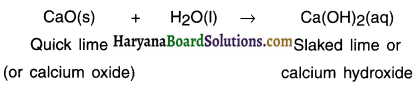Question 2.
Why is the amount of gas collected in one of the test tubes in Activity 1.7 double of the amount collected in the other? Name this gas.
In activity 7. water is electrolysed to give H2 gas at one electrode and O2 gas at the other electrode. The chemical reaction for this is:
2H2O(l) → 4 2H2(g) + O2(g)
Thus, two molecules of water on electrolysis give 2 molecules of hydrogen gas and 1 molecule of oxygen gas. Hence, the amount of hydrogen gas collected will be double than that of oxygen gas.Textbook Page no – 13

Question 1.
Why does the colour of copper sulphate solution change when an Iron nail is dipped In It?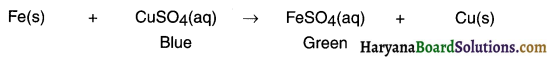Iron is more reactive than copper. Hence, it displaces copper from the solution of copper sulphate. As a result, the blue colour of copper sulphate fades away when it becomes green coloured solution of ferrous sulphate.

Question 2.
Give an example of a double displacement reaction other than the one given in Activity 1.10.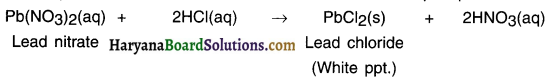PB++ ions from lead nitrate combine with 2Cl ions from hydrochloric acid and a precipitate of lead chloride is formed. Thus, there is an exchange of ions between the two reactants i.e., lead nitrate and hydrochloric acid, and hence this reaction is a double displacement reaction.

Question 3.
Identify the substances that are oxidized and the substances that are reduced in the following reactions.
(i) 4Na(s) + O2(9) → 2Na2O(s)
(i) 4Na(s) + O2(g) → 2Na2O(s)
Na has gained oxygen and forms Na2O.
So, Na is oxidized and O2 is reduced.

(ii) CuO(s) + H2(g) → Cu(s) + H2O(I)
(ii) CuO(s) + H2(g) → Cu(s) + H2O(I)
CuO has lost oxygen and forms Cu.
So, Cu is reduced while H2 has gained oxygen, hence, it is oxidized.Activities

Activity 1.
Perform an activity of burning magnesium ribbon.
1. Clean a magnesium ribbon about 3-4 cm long by rubbing it with sandpaper.
2. Hold it with a pair of tongs. Burn it using a spirit lamp or burner and collect the ash formed in a watch-glass as shown in figure. Burn the magnesium ribbon keeping it away as far as possible from your eyes.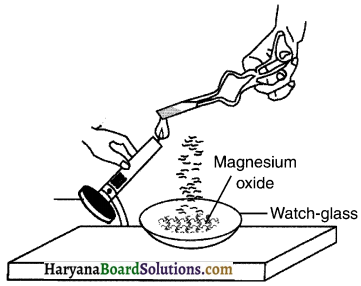Observation:
The magnesium (Mg) ribbon starts burning with a dazzling white flame and changes into white powder. The powder formed is called magnesium oxide (MgO).

Conclusion:
On burning magnesium (Mg) in the presence of oxygen (O2) produces magnesium oxide (MgO) through a chemical reaction between magnesium and oxygen.

Activity 2.
Take lead nitrate solution in a test tube.
Add potassium iodide solution to this. What do you observe?

Observation:
A yellow precipitate of lead iodide is formed.

Conclusion:

Activity 3.
Perform an activity to produce hydrogen gas from zinc.
1. Take a few zinc granules in a conical flask or a test tube.
2. Add dilute hydrochloric acid or sulphuric acid to this.

• Do you observe anything happening around the zinc granules?
• Touch the conical flask or test tube. Is there any change in its temperature?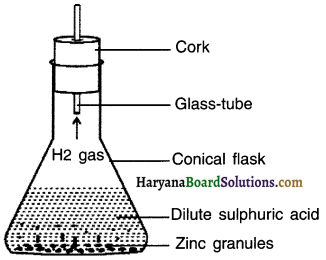Observation:
Bubbles of hydrogen gas get formed around zinc granules.Conclusion:
On adding dilute hydrochloric or sulphuric acid to zinc granules, hydrogen gas is produced. It also increases the temperature of the solution. This shows two characteristics,
(1) Evolution of gas and
(2) Change in temperature.

Activity 4.
Perform an activity with calcium oxide to demonstrate endothermic reaction.
Take a small amount of calcium oxide (quick lime) in a beaker.
Now slowly add water in the beaker.
Touch the beaker.

Observation:
You will observe that the beaker has become hot. This means that the reaction has released heat and hence it is exothermic reaction.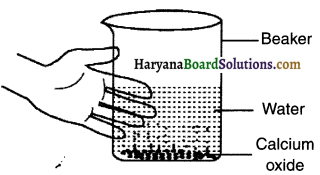Reaction:
When calcium oxide and water are mixed, calcium oxide reacts vigorously with water to produce slaked lime (calcium hydroxide) and release a large amount of heat.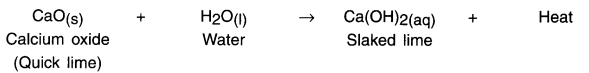Activity 5.
Explain thermal decomposition of ferrous sulphate with suitable diagram and chemical equation.
1. Take about 2g ferrous sulphate crystals in a dry boiling tube.
2. Note the colour of the ferrous sulphate crystals.
3. Heat the boiling tube over the flame of a burner or spirit lamp as shown in figure.
4. Observe the colour of the crystals after heating.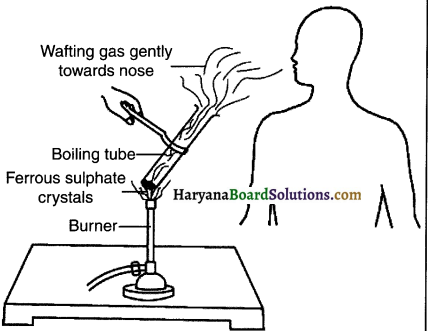Observation:
When ferrous sulphate is heated strongly, it looses water and decomposes to form ferric oxide, sulphur dioxide gas and sulphur trioxide gas.

Reaction: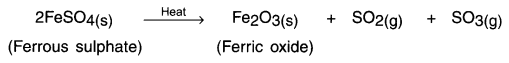The green coloured ferrous sulphate changes to brown coloured ferrous oxide.
The odour of burning sulphur can be smelled in the test tube.

Activity 6.
Perform an activity to show thermal decomposition reaction of lead nitrate.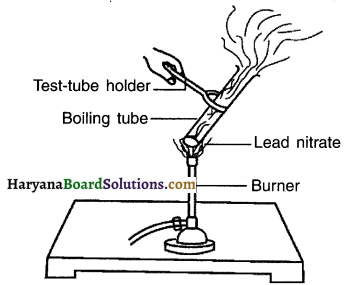Hold the boiling tube with a test-tube holder and heat it over a flame, as shown in figure.

Observation:
On heating lead nitrate, gets decomposed to produce lead oxide nitrogen dioxide and oxygen.
The reaction emits brown fumes of nitrogen dioxide.

Reaction: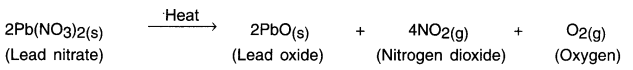Electrical decomposition/Electrolysis of water

Activity 7.

Explain electrolysis of water through electrical Decomposition
Take a plastic mug. Drill two holes at its base and fit rubber stoppers in these holes. Insert carbon electrodes in these rubber stoppers as shown in figure.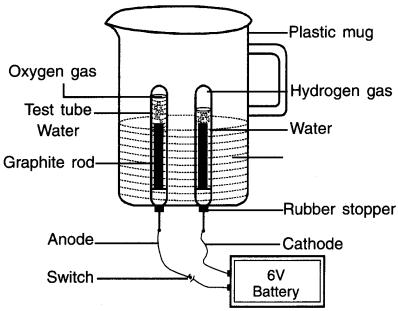1. Connect these electrodes to a 6-volt battery.
2. Fill the mug with water such that the electrodes get immersed. Add a few drops of dilute sulphuric acid to the water.
3. Take two test tubes filled with water and carefully invert them over the two carbon electrodes.
4. Switch on the current and leave the apparatus undisturbed for some timer
5. You will observe the formation of bubbles at both the electrodes. These bubbles are formed by decomposition of water on passing electricity.

Observation:
1. On passing electricity, the water decomposes into hydrogen gas and oxygen gas. The formation of bubbles at both the electrodes is due to these gases.

2. As can be seen, more gas is produced on the test-tube on the right hand side. This is hydrogen gas. The hydrogen gas is produced in double volume as compared to oxygen produced in the left test tube.

Testing the presence of gases:

•  Take out the test-tube on the left side i.e. the one which contains less gas and bring it near a burning candle. You will see that the gas burns brightly. This means the gas is oxygen.
• Similarly, the gas in the right test-tube burns with a popping sound which means it is hydrogen gas.Activity 8.
Perform an activity to demonstrate decomposition through light. OR Perform and activity to show the decomposition of
silver chloride
1. Take about 2g silver chloride (AgCI) in a china dish. Observe its colour.
2. Place the china dish under sunlight for some time. Again observe its colour.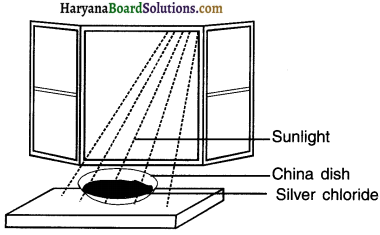Observation:
Initially the colour of silver chloride was white. After keeping it under sunlight for some time its Fig colour changed to grey.

Reaction:
When white coloured silver chloride is kept under sunlight it gets decomposed and produces white coloured silver metal and chlorine.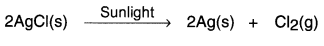Activity 9.
Perform an activity to show the displacement of copper.
1. Take three iron nails and clean them by rubbing with sand paper.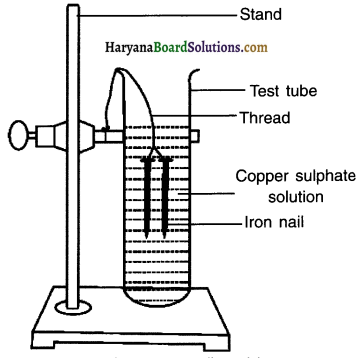2. Take two test tubes marked as (A) and (B). In each test tube, take about 10 mL copper sulphate solution.

3. Tie two iron nails with a thread and immerse 04: them carefully In the copper sulphate solution in test tube B for about 20 minutes. Keep one iron nail aside for comparison.

4. After 20 minutes, take out the iron nails from the copper sulphate solution.Question 1.
Compare the intensity of the blue colour of copper sulphate solutions in test tubes (A) and (B).
Observation:
The colour of copper sulphate in test-tube A is Figur blue. The blue colour of copper sulphate fades in test-tube B and the solution becomes light blue.

Question 2.
Also, compare the colour of the iron nails dipped in the copper sulphate solution with the one kept aside [Fig. 1.8 (b).
Observation:
iron nail dipped in copper-sulphate solution becomes shinning brown as compared to an ordinary iron nail.

Reaction: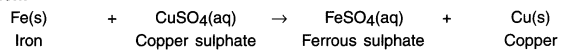Conclusion:
Iron is a more reactive element. So, it displaces copper from copper sulphate solution.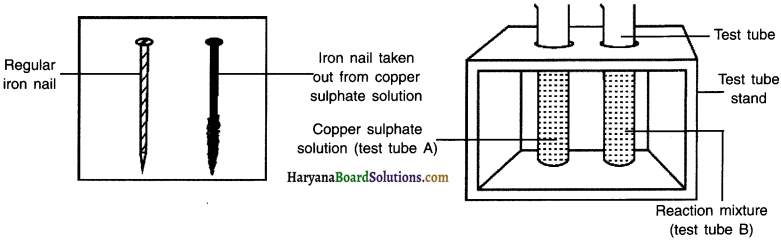(b) Iron nails and copper sulphate solutions compared before and after the experiment.

Activity 10.
Perform an activity to show double displacement reaction.
1. Take about 3 mL of sodium sulphate solution in a test tube.
2. In another test tube, take about 3 mL of barium chloride solution.
3. Mix the two solutions.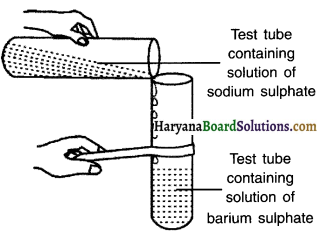Observation:
1. When aqueous solution of sodium sulphate reacts with an aqueous solution of barium chloride, a white precipitate of BaSO4 is formed.
2. The precipitate is insoluble whereas the other product i.e. sodium chloride remains in the solution.
Reaction: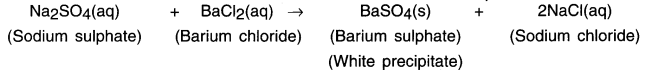Activity 11.
Perform an activity to study oxidation reaction.